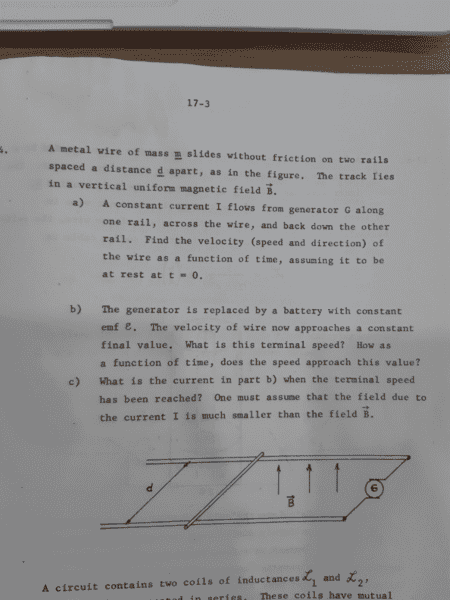# The speed of a metal wire on two rails with a magnetic field

## Homework Statement

I tried to understand the problem b) and c).[/B]## Homework Equations

Faraday's law: ∇xE = - ∂B/∂t
emf ε = Bdv
Force : F =ma, Lorenz's force F=q(vxB) ==> ma = IdB
Power : power of battery = εI, mechanical power of the wire = Fv

## The Attempt at a Solution

I think I solved a), where the magnetic force on the wire is F = IdB = ma and the speed v = at = IdBt/m.
I'm now tried to understand b) and c). First, I assume the initial speed is 0 at t=0 when the generator is replaced by a battery. The battery's power is εI, and it must be equal to the power consumed by the wire which is Fv = IdBv. Then the speed of the wire is v = ε/dB.
Also using Faraday's law I get to the same conclusion : ∇xE = - ∂B/∂t >>> ε = -∂(flux)/∂t = Bdv
It doesn't feel natural. The battery is connected at t=0, and in no time there the wire goes at speed v=ε/dB? So I think it is nonsense. But I don't know where I choose a wrong way. Help me plz.
I guess the answer to c) is that the current has to be 0, because when the terminal speed has been reached, there is no acceleration, then no force F=IdB=0. Am I right at this?

#### Attachments

kuruman
Homework Helper
Gold Member
2021 Award
In part (a), you cannot use ##v=at## because the acceleration is not constant. As the bar accelerates, the current ##I## is also not constant, but depends on the velocity which depends on time. You need to solve a differential equation for part (a). Start with Newton's 2nd Law in the form $$m\frac{dv}{dt}=IB~d$$and then use Faraday's Law to find ##I## in terms of ##v##. Put that back in Newton's 2nd Law and solve.
Fv = IdBv. Then the speed of the wire is v = ε/dB.
How do you figure? The variable "v" cancels out in the equation.

On edit: Ooops! I meant part (b) in what I wrote above, not part (a). Part (a) is correct because the rails are connected to a constant current generator.

Last edited:
Perhaps this thread is similar to (b) and (c) questions in your problem:
https://www.physicsforums.com/threa...-inside-a-magnetic-field.952728/#post-6036839
In your problem (questions (b) anc (c)) there are a force due the constant potential ##\varepsilon##. In the above link the force is due to a constant gravitational potential near the Earth surface. Is a little bit different but I think above thread can help you.

kuruman, I've have been thinking about this problem from yesterday because it is hard. I don't know if in the (a) question there are a variable electric potential that compensates ##\varepsilon_inducc## and then Intensity really is a constant. I don't know.
Edit.
Also it is important the direction of circulation of Intensity, because the bar must be moved to the left in the picture.

kuruman
Homework Helper
Gold Member
2021 Award
kuruman, I've have been thinking about this problem from yesterday because it is hard. I don't know if in the (a) question there are a variable electric potential that compensates εinducc\varepsilon_inducc and then Intensity really is a constant. I don't know.
I retracted my statement on edit. Part (a) is correct.
Also it is important the direction of circulation of Intensity, because the bar must be moved to the left in the picture.
Why? The direction of the field is shown in the figure, but without knowing which way the battery is connected, one cannot figure out the direction of the velocity.

I says about the direction o velocity because the diagram, if the bar moves to the right it will crashes whit the generator. Ok. if the supports wires are so long this is not important.

knowone needs to calculate ##\varepsilon_{inducc}## that is related with ##v## (when he applies Faraday's Induction law). After that I think that he can try:
$$\varepsilon -\varepsilon_{inducc}=I·R$$
Then knowone can get Intensity, and put it into the movement equation that you has wrote above.
Then he will need to solve the differential equation.
Please if there are some mistake say me it. In my work problems do not are so hard.

kuruman
Homework Helper
Gold Member
2021 Award
Then knowone can get Intensity, and put it into the movement equation that you has wrote above.
Then he will need to solve the differential equation.
Please if there are some mistake say me it. In my work problems do not are so hard.
Your reasoning is correct. Let's wait and hear what @knowone has to say.

How do you figure? The variable "v" cancels out in the equation.

The power of the battery is εI and it equals Fv=IdBv. Thus εI=idBv and v= ε/dB

I solved these equations:
εbatteryinduced =IR, εinduced = vdB, and m(dv/dt)=IBd
Then I= (εbattery-vdB)/R, thus m(dv/dt)=Bd((εbattery-vdB)/R).

Using differential equation, v(t)=A(1-e-λt), where A = -εbattery/d2B2 and λ = d2B2/mR

Here we added a arbitrary constant R. I'm reluctant to use it because there is no specification about any resistance of the circuit.

If we are allowed to use the solution v(t), then what will be the time when the system arrives at equilibrium?

Last edited:
kuruman